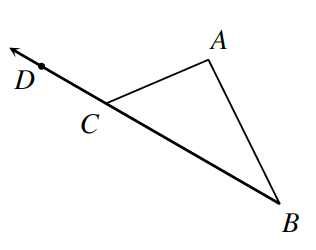### Home > GC > Chapter 7 > Lesson 7.2.2 > Problem7-59

7-59.In the diagram at right, $∠DCA$ is referred to as an exterior angle of $ΔABC$ because it lies outside the triangle and is formed by extending a side of the triangle.

1. If $m∠CAB=46°$ and $m∠ABC=37°$, what is $m∠DCA$? Show all work.

How do the angles of a triangle relate to each other? How will this help you solve for $∠DCA$?

$46°+37°+∠ACB=180°$ Then how are $∠ACB$ and $∠ACD$ related?

2. If $m∠DCA=135°$ and $m∠ABC=43°$, then what is $m∠CAB$

Use similar methods to those used for part (a). The methods might be used in a different order.